Peripheral nerve magnetic stimulation: influence of tissue non-homogeneity

Abstract

Background

Peripheral nerves are situated in a highly non-homogeneous environment, including muscles, bones, blood vessels, etc. Time-varying magnetic field stimulation of the median and ulnar nerves in the carpal region is studied, with special consideration of the influence of non-homogeneities.

Methods

A detailed three-dimensional finite element model (FEM) of the anatomy of the wrist region was built to assess the induced currents distribution by external magnetic stimulation. The electromagnetic field distribution in the non-homogeneous domain was defined as an internal Dirichlet problem using the finite element method. The boundary conditions were obtained by analysis of the vector potential field excited by external current-driven coils.

Results

The results include evaluation and graphical representation of the induced current field distribution at various stimulation coil positions. Comparative study for the real non-homogeneous structure with anisotropic conductivities of the tissues and a mock homogeneous media is also presented. The possibility of achieving selective stimulation of either of the two nerves is assessed.

Conclusion

The model developed could be useful in theoretical prediction of the current distribution in the nerves during diagnostic stimulation and therapeutic procedures involving electromagnetic excitation. The errors in applying homogeneous domain modeling rather than real non-homogeneous biological structures are demonstrated. The practical implications of the applied approach are valid for any arbitrary weakly conductive medium.

Background

The analysis of electrical fields induced by magnetic stimulation has been addressed by many authors. However, possible domain non-homogeneity, non-linearity, or anisotropy usually have not been considered, even in relatively recent works . However, some studies have to some extent taken into account media characteristics , and Miranda et al.  did consider non-homogeneity in brain tissue layers. Non-homogeneities do influence correct coil design, excitation current, and positioning with respect to stimulation sites.

It is well known that excitation of peripheral nerves is achieved by electrical current or magnetically induced current, especially by the component parallel to the nerve, as ensuing from the well-known cable equation :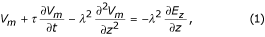where V m , τ, λ, are, respectively, the transmembrane potential, the membrane time-constant, and the fiber length constant.

With magnetic stimulation, the Jz component, only a fraction of the globally induced current field pattern, is associated with the properties of the conductive medium. The induced current density (J) distribution depends on the electric field, but also on the tissue specific resistivities. Excitation will occur at sites where the local current density exceeds a certain threshold.

The cross-section of the wrist region anatomical structure is shown in Fig. 1. The induced fields should be assessed by modeling, taking into account the real characteristics of this magnetically homogeneous and markedly electrically non-homogeneous structure. Constructing a model based on homogeneous medium only would lead to inaccurate results. Assessment of the possible errors involved with such a model requires special investigation, which exceeds the aims of this report.

When diagnosing, for example, nerve compression in the carpal canal by using magnetic stimulation, one difficulty is the need for separate excitation of the median and ulnar nerves. The electromyogram examination for diagnosis of nerve compression in the carpal canal is done routinely by electrical stimulation of n. medianus over the wrist, and recording the evoked potential from the 3rd finger (the 4th finger is also partly innervated by n. medianus). More proximal stimulation is not recommended, especially for the diagnosis of carpal tunnel syndrome, as other injury may be present along the nerve.

The problem of separate electrical stimulation of n. medianus and n. ulnaris is especially complicated in babies and small children, due not only to the small size of the member, but also to the intensive pain involved.

From a biomedical engineering point of view, the problem is related to the induced electrical field and the resulting current density distribution analysis in non-homogeneous and anisotropic biological structures.

The above considerations defined our main study objectives :

• to show and assess the differences between induced fields and current density with and without accounting for non-homogeneity, using an adequate model of the stimulated object;

• to assess the possibility of selective median and ulnar nerve stimulation in the wrist region.

Methods

A 3D model of the carpal region was constructed (Fig. 2), simulating the non-homogeneous anatomical structure (maximum diameter 7 cm, length 4 cm), using ANSYS 5.7 (Ansys, Inc., Houston, USA). For the purpose of simplifying the finite element model (FEM) mesh structure, some regions of approximately equal conductivity were joined in common sub-areas, as seen by comparing Fig. 1 and Fig. 2. The 3D model was obtained by translation of a 2D section, obtaining a quasi-cylindrical domain with 4 cm generatrix. This dimension was considered to be anatomically acceptable.

In addition, this study involved the use of MATLAB 5.2 (MathWorks, Inc., Natick, USA), Mathematica 4.0 (Wolfram Research, Inc., Champaign, USA) and several in-house developed linking modules.

The designed model consisted of 20,520 nodes and 114,638 'solid 97' elements. The software automatically selects one of four options, depending on the sub-regions structure (Fig. 2). Two model versions were considered, allowing assignment of different resistivities to the respective regions, as follows:

1. a)

homogeneous structure of specific resistivity ρ = 5 Ωm; the value was chosen as a very approximate average of the conductivity of nerves.

2. b)

non-homogeneous structure that takes into account the anisotropy of the nerves and the muscles. The specific resistivities were chosen to correspond to the regions marked in Fig. 2, as follows [e.g. [6, 11]]:

M 1 – connective tissue, buffer zone between various tissues - ρ1 = 10 Ωm;

M 2 – tendons - ρ2 = 5 Ωm;

M 3 – extracellular space - ρ3 = 6 Ωm;

M 4 – blood vessels - ρ4 = 2.5 Ωm;

M 5 – nerves - ρ5x = ρ5y = 10 Ωm ; ρ5z = 1 Ωm;

M 6 – bone - ρ6 = 160 Ωm;

M 7 – cartilage - ρ7 = 40 Ωm;

M 8 – skin and fat - ρ8 = 20 Ωm;

M 9 – articular disc - ρ9 = 60 Ωm;

M 10 – muscles - ρ10x = ρ10y = 13.2 Ωm; ρ10z = 1.9 Ωm;

The medium was taken to be magnetically homogeneous, with relative magnetic permeability μ r = 1 assigned to all sub-regions.

The external magnetic field was excited by five identical square-shaped coils (1 cm side) in fan-like configuration (slinky coils ), positioned 5 mm above the skin (due to the need for adequate coil isolation). The 1 cm active coil length was chosen taking into account the model length, limited to ± 2 cm, and also the fact that although of small size, such coils can be manufactured.

The excitation currents for the coils were generated by RLC-contour capacitor discharge (R = 1.75 Ω, L = 5.146 μH, C = 32 μF), with I = 1000A (peak current). The initial current slope (di(t) / dt) t=0was assessed to be 107 A/s, at f = 10 kHz approximate equivalent frequency in stationary sinusoidal mode. The influence of skin, proximity and twisting effects  were not considered in the computations for R and L.

Two coil positions were studied (Fig. 2): (A) for stimulation of the median nerve, and (B) for stimulation of the ulnar nerve. The same type of coordinate system was used for each of the coil positions: Z-axis along the quasi-cylindrical surface generatrix (i.e. parallel to the nerves), Y-axis perpendicular to the surface, and X-axis tangent to the surface. Z = 0 was selected at the center of the common part of the coils.

The coil disposition (Fig. 2) with respect to the two nerves yielded the following distances (from the center of the active conductor to the center of the respective nerve): from coil A to the median nerve 13,5 mm and to the ulnar nerve 40 mm; from coil B to the median nerve 38 mm and to the ulnar nerve 18,3 mm.

The analysis of the induced eddy fields in the non-homogeneous domain was performed according to a previously developed approach . Its main points are:

a) Defining the field of the external electromagnetic source by the equation for the magnetic vector potential of a current contour [e.g. ]:For a number of n coils, each of w windings, and currents i k (t), k = 1,2,...,n, the following equation was used:b) Calculation of the three vector-potential components A x , A y and A z for the nodes which belong to the boundary regions of the examined 3D volume (1900 nodes). These potentials were introduced as Dirichlet boundary conditions.

The relation forin 3D medium, taking into account non-homogeneity and for a region without internal current sources, can be presented as [e.g. [14, 15]]: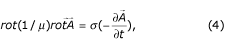For magnetically homogeneous media (μ = const), using the Coulomb gauge div= 0 and neglecting the field potential component φ, the homogeneous form of the diffusion equation is obtained:For sinusoidal harmonic potentials the Helmholtz equation was used:where σ = 1/ρ is the specific conductivity of the respective region.

The strict solution of the problem for φ ≠ 0 and div= 0, neglecting the current of the electrical induction, requires the use of a system of equation [14, 16]: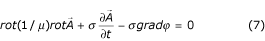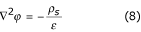In case of non-homogeneous medium with small differences between specific conductivities, the surface charges ρ s at the boundaries are neglected  and respective φ ≡ 0.

c) The boundary conditions at the interface between different media are obtained automatically by the FEM , in connection with the principles of continuity, ensuing from the Maxwell equations.

d) The induced electrical field vector in homogeneous sub-regions is determined in the FEM by the following relations: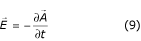or in the harmonic mode: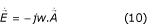The respective current density is: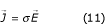Results

The boundary conditions for the magnetic vector potential(x, y, z, t), applied to the exterior nodes of the 3D model, were determined from Eq. (3), using the selected peak current value I = 1000 A. The solutions of Eq. (3) for stimulation coils in fan configuration, located at selected basic positions (A) and (B) of the model, are presented in Figs. 3(a) and 3(b), respectively.

Using the software for FEM modeling, harmonic magnetic analysis was performed to obtain the eddy current distribution in the medium. Figures 4,5,6,7 represent the current distribution profiles in the medium (a) and separately in the nerves (b), studied for both coil dispositions – (A) (Fig. 4, 5) and (B) (Figs. 6, 7), each examined in two versions – homogeneous and non-homogeneous anisotropy for the nerve and muscle region.

As was noted in the Background section above, excitation of peripheral nerves is achieved by electrical current or magnetically induced current, especially by the current component parallel to the nerve. The induced current density distribution (Jz) depends on the electric field, but also on the tissue specific resistivities. Excitation will occur at sites where the local current density exceeds a certain threshold.

The results for the current density component Jz in the nerves are summarized in Table 1. The parameter "Ratio Jz max 10%" rates how many times the current density in the stimulated nerve was higher than J z in the opposite nerve, estimated over 10% of the elements with maximum J z . This parameter is used to measure the possibility and quality for selective stimulation.

The graphs of the eddy current distribution profile J = J(z) along a line parallel to the nerve fiber axis Z, chosen to include the elements with higher current density, are shown in Fig. 8. The subplots Fig. 8 (a-d) correspond to the four cases presented in Table 1. Following Ohm's law in differential form (Eq. 11), data for the electric field distribution profile E = E(z) can be directly obtained using the nerve specific resistivity along the Z-axis: ρ z = 1/σ z = 1 Ωm. The distribution of the eddy current vector is shown in Fig. 9.

Discussion

We should note that the image definition (Figs. 4,5,6,7) and the current profiles smoothness (Fig. 8) depend on the finite element mesh size used, which was restricted by the total number of elements in the model and their respective distribution (Fig. 2).

The impact of considering non-homogeneity and anisotropy in this particular task can be assessed by comparing the respective current density distributions in Figs. 4,5 and Figs. 6,7. The eddy currents in the non-homogeneous model are concentrated in low resistivity regions under the stimulation coils. Moreover, the maximum eddy current density in the nerves was found to be significantly higher in the case of non-homogeneous domain – about 4.31 times for the median nerve and 4.91 times for the ulnar nerve (Table 1). The selected average value of the homogeneous domain specific resistivity (ρ = 5 Ωm) is lower than that of the compound structure, where the bone (ρ = 160 Ωm) and other tissues (ρ > 5 Ωm) occupy more than about 33% and 25% of the domain, respectively. The blood vessels, having much lower specific resistivity, cover negligibly smaller portion of the domain.

Another way to present the inadequacy of homogeneous analysis can be seen in the graphs of Fig. 8. As shown above, J z for the coil position (A) in the non-homogeneous domain has four to five times higher peak value than in the homogeneous medium. The current density is not exactly proportional to the conductivity because current density distribution is considered – J will depend on conductivity of adjacent structures due to compression or rarefaction of current lines. These results might be useful for future research related to stimuli propagation along the nerve.

In connection with the profiles of Fig. 8, it should be noted that at every node of the boundary surfaces the value of the vector-potential was obtained from the coil current. The eddy currents induced were defined by solving this boundary problem with dependence of the specific resistivities in each volume element and of the accepted equivalent frequency. Jz is therefore not restricted to a condition Jz = 0 at the boundaries, so that the profiles obtained might be accepted as realistic. Also, the model length of ± 2 cm compared to the 1 cm active coil side seems to be an acceptable compromise.

The possibilities for selective stimulation were assessed. The distances between coils and nerves should be taken into account. As specified in Methods section above, these distances are (Fig. 2): from coil position A to the median nerve 13,5 mm, and to the ulnar nerve 40 mm; from coil position B to the median nerve 38 mm and to the ulnar nerve 18,3 mm.

The selectivity was estimated by the parameter "Ratio" (see Table 1). The coil position (A) results in induction of current density in the stimulated median nerve that is 8 to 12.5 times higher than in the ulnar nerve. The opposite position (B), for ulnar nerve stimulation leads to lesser selectivity – the induced current density is 2 to 3 times higher than for the median nerve.

Considering the proposed model, some specific comments can be made.

1. 1.

The relatively complicated introduction of stimulation coil currents in the basic FEM software module was avoided. A first step involved an analytical-numerical procedure for obtaining the magnetic vector-potential of the external field. The necessary computation of the integrals in Eq. (3) does not lead to essential difficulties.

2. 2.

The solution of the internal Dirichlet problem, after the already available vector-potential of the external field and its use for defining the boundary conditions, becomes a routine procedure. According to the uniqueness theorem [e.g. [17, 18]], the solution was unique, considering the limited volume. The accepted non-homogeneity did not infringe on this condition [14, 15]. An indirect verification for respecting the uniqueness conditions, even with the introduced partial anisotropy, was the relatively fast convergence of the procedures related to the FEM application.

3. 3.

The program allowed solution of the Helmholtz equation, valid for harmonic mode. The solution for harmonic mode could lead to unstable solutions using FEM, even for analysis in linear domains, depending on various factors (e.g. geometry of the region and sub-regions, type of mesh generator, elements used, etc). The proposed approach yielded stable solutions in all cases.

4. 4.

A more accurate model would require increasing the domain longitudinal dimension while preserving solution stability. Increasing the length from 4 to 6 cm would raise the number of elements from 114,638 to 180,000. A still more detailed model structure would require more than 600,000 elements.

5. 5.

The excitation system selected, with five fan-like square coils, was one possible application. Optimization procedures could be used, with appropriate criteria and limiting conditions. The selected coil size is rather small, but it is feasible and consistent with the model (and wrist) dimensions.

6. 6.

Additional studies may include the cable equation (Eq. 1), in relation to propagation velocities along peripheral nerves, by taking into account non-homogeneity and anisotropy, for various coil shapes and positions.

7. 7.

The proposed procedure is applicable, in principle, for electromagnetic stimulation of other excitable structures.

Conclusion

We have presented an application of the induced electrical field approach to magnetic stimulation of peripheral nerves in non-homogeneous tissues. Solutions of two specific problems were proposed:

• assessment of the induced electrical field gradient under conditions of non-homogeneity and in relation to possible solution of the cable equation;

• analysis of the possibilities for selective stimulation.

The method developed is of limited accuracy, but its possible errors should be considered in view of the various random factors appearing in the process of stimulation. The results presented demonstrate that neglecting non-homogeneity and to some extent anisotropy, could introduce essential and strongly misleading errors.

References

1. 1.

Basser P, Roth B: New currents in electrical stimulation of excitable tissues. Annu Rev Biomed Eng 2000, 02: 377–397. 10.1146/annurev.bioeng.2.1.377

2. 2.

Tofts PS: The distribution of induced current in magnetic stimulation of the nervous system. Phys Med Biol 1990, 35: 1119–1128. 10.1088/0031-9155/35/8/008

3. 3.

Ruohonen J, Ravazzani P, Nilsson J, Panizza M, Grandori F, Tognola G: A volume-conduction analysis of magnetic stimulation of peripheral nerves. IEEE Trans Biomed Eng 1996, 43: 669–678. 10.1109/10.503174

4. 4.

Karu PE, Stuchly MA: Quasi-static electric field in a cylindrical volume conductor induced by external coils. IEEE Trans Biomed Eng 1994, 41: 151–158. 10.1109/10.284926

5. 5.

Roth BJ, Saypol JM, Hallet M, Cohen LG: A theoretical calculation of the electric field induced by magnetic stimulation of a peripheral nerve. Muscle & Nerve 1994, 13: 734–741.

6. 6.

Nagarajan S, Durand M: Analysis of magnetic stimulation of a concentric axon in a nerve bundle. IEEE Trans Biomed Eng 1995, 42: 926–932. 10.1109/10.412659

7. 7.

Roth BJ, Saypol JM, Hallet M, Cohen LG: A theoretical calculation of the electric field induced in the cortex during magnetic stimulation. Electroenceph Clin Neurophysiol 1991, 81: 47–56. 10.1016/0168-5597(91)90103-5

8. 8.

Ragan PM, Wang W, Eisenbrg SR: Magnetically induced currents in the canine Heart: A finite element study. IEEE Trans Biomed Eng 1995, 42: 1110–6. 10.1109/10.469378

9. 9.

Krasteva VT, Papazov SP, Daskalov IK: Magnetic Stimulation for non-homogeneous biological structures. BioMed Eng Online 2002, 1: 3. 10.1186/1475-925X-1-3

10. 10.

Miranda PC, Hallet M, Basser PJ: The electric field induced in the brain by magnetic stimulation: A 3D Finite-element analysis of the effect of tissue heterogeneity and anisotropy. IEEE Trans Biomed Eng 2003, 50: 1074–1085. 10.1109/TBME.2003.816079

11. 11.

Malmivuo J, Plonsey R: Bioelectromagnetism. Oxford University Press, New York-Oxford 1995.

12. 12.

Ravazzani P, Ruohonen J, Tognola G, Anfonsso F, Ollikainen M, Ilmoniemi , Grandori F: Frequency-related effects in the optimization of coil for magnetic stimulation of nervous system. IEEE Trans Biomed Eng 2002, 49: 463–470. 10.1109/10.995685

13. 13.

Panofsky WKH, Phillips M: Classical electricity and Magnetism. Addison-Wesley Publishing Company, INC, Cambridge 42, Mass 1964.

14. 14.

Coulomb J-L, Sabonnadiere J-C: Cao en Electrotechnique. Hermes ublishing 1985.

15. 15.

Silvester PP, Ferrari RL: Finite elements for electrical engineers. Cambridge University Press Cambridge-London-New York 1983.

16. 16.

Novozhilov YuV, Yappa YuA: Electrodynamics. Mir Publishers, Moscow 1981.

17. 17.

Jakson JD: Classical Electrodynamics. JW & Sons, New-York-London 1962.

18. 18.

Stratton JA: Electromagnetic theory. McGGRAW-HILL, New York and London 1941.

Acknowledgements

The authors thank the Technical University of Sofia for granting use of the FEM software.

Author information

Correspondence to Ivan K Daskalov.

Authors' contributions

Author SP was responsible for the theoretical basis of the investigation. Author VK solved the problems of adequate FEM modeling and carried-out the generation and presentation of various field and current distributions. Author ID was responsible for setting and formulation of the problem, directing the research, and the biomedical engineering aspect of the study. All authors read and approved the final manuscript.

Authors’ original submitted files for images

Below are the links to the authors’ original submitted files for images.

Rights and permissions

Reprints and Permissions